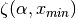Warning

This documents an unmaintained version of NetworkX. Please upgrade to a maintained version and see the current NetworkX documentation.

# zipf_rv¶

zipf_rv(alpha, xmin=1, seed=None)[source]

Return a random value chosen from the Zipf distribution.

The return value is an integer drawn from the probability distribution ::math:

p(x)=\frac{x^{-\alpha}}{\zeta(\alpha,x_{min})},


whereis the Hurwitz zeta function.

Parameters: alpha (float) – Exponent value of the distribution xmin (int) – Minimum value seed (int) – Seed value for random number generator x – Random value from Zipf distribution int ValueError – If xmin < 1 or If alpha <= 1

Notes

The rejection algorithm generates random values for a the power-law distribution in uniformly bounded expected time dependent on parameters. See  for details on its operation.

Examples

>>> nx.zipf_rv(alpha=2, xmin=3, seed=42)


References

.. Luc Devroye, Non-Uniform Random Variate Generation,
Springer-Verlag, New York, 1986.